# Thursday October 5th

## PureScript Night recap

• Tuesday night, we had our first PureScript Meetup!

## JS issues … why PureScript

• scaling up/ getting up to speed with a large code-base is often difficult
• PureScript produces minimal and easily readable JS
• it doesn’t contain bloated libraries; you use more of only what you need
• it has a fast runtime system
• strong expressive types
• there is more predictability of code from PureScript to JS

## Why he wrote a language vs taking it off the shelf

• TypeScript/Flow vs PureScript
• real row polymorphism from type checker
• Haste/Fay
• Fay didn’t include typeclasses support
• GHCJS was not easy to read for JS team
• didn’t have runtime
• Roy (programming language) was the closest that satisfied what he wanted

## Resources

• Pursuit - PureScript documentation (built with Yesod)
• PureScript Contrib
• PureScript Node
• PureScript web
• Core libraries
• !!YPER (server side rendered), uses type system to enforce certain things
• Pux (for front end applications)
• PureScript Halogen (100% PureScript)

## Applications

• Slamdata has 100% PureScript on front end (developed Halogen)
• Quicktype -IDL
• Insect (a personal favourite of mine! )

## Getting Started

• install pureScript contrib
• most people use `pulp`
• book (PureScript by example)
• Getting started with PureScript (pureScript documentation)

## Future Work

• ApplicativeDo
• SAT Solvers (constraints/ solutions)
• parsing JSON
• argonaut
• simple generics

## CodeGen talk

• Brady did a talk on using CodeGen in PureScript from Haskell Types

## After Meetup-hangout

• We went out for drinks afterwards at the Arts District Brewery. It was really fun! Phil was also kind enough to give two of us a ride home. :) I have a lot of great expectations for this group!

## This led to my writing my first PureScript programme!

• I started a repo with such projects, as I continue attending the Meetup and work on PureScript on my own.

## Easy to get set up

• It’s surprisingly easy to get up and running with PureScript. Here is checking the type of “map”.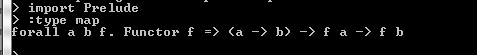• They have you do Euler question number 1. I’ve done it in Python before, so that was my comparison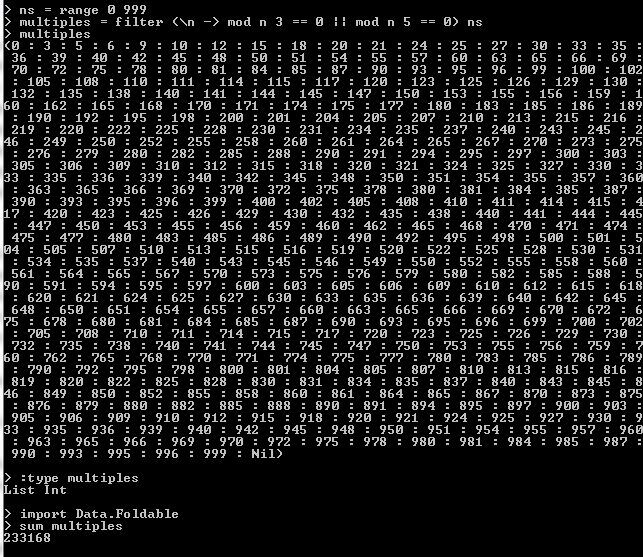• The prompt upon leaving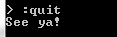• Obligatory “Hello World” in PureScript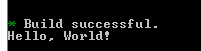• installing pureScript-global and browsing the module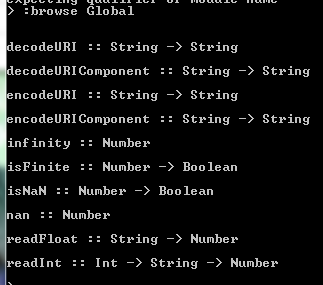• solution for my exercise from my first programme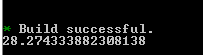## Katas

• Find number of non-even substrings in a string integer
``````def solve(s):
arr = []
import itertools
a = (s[i:j] for i, j in itertools.combinations(xrange(len(s)+1), 2))
for i in a:
if int(i) % 2 != 0:
arr.append(i)
return len(arr)
``````
• Find number in word and return its integer equivalent
``````function findNum(n){
result = 0
n = n.toLowerCase()
var a = n.replace(/[^\w\s!.]/g,'');
var b = a.replace(/ /g,'')
for (var i = 0; i < b.length; i++){
if (b.indexOf('one') !== -1){
result = 1
}
else if (b.indexOf('two') !== -1){
result = 2
}
else if (b.indexOf('three') !== -1){
result = 3
}
else if (b.indexOf('four') !== -1){
result = 4
}
else if (b.indexOf('five') !== -1){
result = 5
}
else if (b.indexOf('six') !== -1){
result = 6
}
else if (b.indexOf('seven') !== -1){
result = 7
}
else if (b.indexOf('eight') !== -1){
result = 8
}
else if (b.indexOf('nine') !== -1){
result = 9
}
else if (b.indexOf('ten') !== -1){
result = 10
}
}
return result
}
``````
• take an array, sort it in desc order and add liftoff essentially
``````function liftoff(instructions){
instructions = instructions.sort((a, b) => a - b);
var a = instructions.reverse()
var b  = a.join(' ') + ' liftoff!'
return(b)
}
``````
• collatz conjecture..count how long it takes number to reach it…
``````def get_collatz(n):
#print n
count = 0
while (not n == 1):
if n % 2 == 0:
n = n / 2
count = count + 1
else:
n = (3 * n) + 1
count = count + 1
return count
``````
• return day given digit eg 1 == Monday, etc
``````function whatday(num) {
if ((num) == 1){
return 'Sunday'
}
else if ((num == 2)){
return 'Monday'
}
else if ((num == 3)){
return 'Tuesday'
}
else if ((num == 4)){
return 'Wednesday'
}
else if ((num == 5)){
return 'Thursday'
}
else if ((num == 6)){
return 'Friday'
}
else if ((num == 7)){
return 'Saturday'
}
else{
return 'Wrong, please enter a number between 1 and 7'
}
``````
• check if string includes ‘tree fiddy’ or ‘3.50’ or ‘three fifty’
``````function isLockNessMonster(s) {
var a = 'tree fiddy'
var b = '3.50'
var c = 'three fifty'

if (s.includes(a) || s.includes(b) || s.includes(c)){
return true
}
else{
return false
}
return
}
``````
• Armstrong number. If for each digit raised to pow 3, total/sum is the number, return true
``````function armstrong(num){
var a = num.toString()
var b = a.split("")
var total = 0
for (var i = 0; i < b.length; i++)
{
var c = Math.pow(parseInt(b[i]), 3)
total = total + c
}
if (total == num){
return(true)
}
else{
return(false)
}
return
}
``````
• turn num to binary string
``````def int_to_bin(x):
z = str(bin(x));
return z[2:]
``````
Written on October 5, 2017